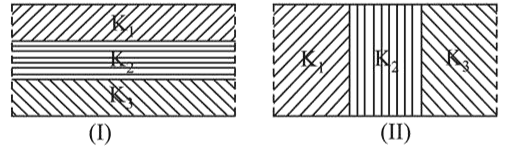# Two identical parallel plate capacitors, of capacitance $C$ each, have plates of area A,

Question:

Two identical parallel plate capacitors, of capacitance $C$ each, have plates of area A, separated by a distance $d$. The space between the plates of the two capacitors, is filled with three dielectrics, of equal thickness and dielectric constants $\mathrm{K}_{1}, \mathrm{~K}_{2}$ and $\mathrm{K}_{3}$. The first capacitors is filled as shown in Fig. I, and the second one is filled as shown in Fig. II.

If these two modified capacitors are charged by the same potential V, the ratio of the energy stored in the two, would be ( $E_{1}$ refers to capacitors (I) and $E_{2}$ to capacitors (II) :1. (1) $\frac{E_{1}}{E_{2}}=\frac{K_{1} K_{2} K_{3}}{\left(K_{1}+K_{2}+K_{3}\right)\left(K_{2} K_{3}+K_{3} K_{1}+K_{1} K_{2}\right.}$

2. (2) $\frac{E_{1}}{E_{2}}=\frac{\left(K_{1}+K_{2}+K_{3}\right)\left(K_{2} K_{3}+K_{3} K_{1}+K_{1} K_{2}\right.}{K_{1} K_{2} K_{3}}$

3. (3) $\frac{E_{1}}{E_{2}}=\frac{9 K_{1} K_{2} K_{3}}{\left(K_{1}+K_{2}+K_{3}\right)\left(K_{2} K_{3}+K_{3} K_{1}+K_{1} K_{2}\right)}$

4. (4) $\frac{E_{1}}{E_{2}}=\frac{\left(K_{1}+K_{2}+K_{3}\right)\left(\mathrm{K}_{2} K_{3}+K_{3} K_{1}+K_{1} K_{2}\right.}{9 K_{1} K_{2} K_{3}}$

Correct Option: , 3

Solution:

(3) $\frac{1}{C_{1}}=\frac{d / 3}{k_{1} \varepsilon_{0} A}+\frac{d / 3}{k_{2} \varepsilon_{0} A}+\frac{d / 3}{k_{3} \varepsilon_{0} A}$

or $\quad C_{1}=\frac{3 k_{1} k_{2} k_{3} \varepsilon_{0} A}{d\left(k_{1} k_{2}+k_{2} k_{3}+k_{3} k_{1}\right)}$

$C_{2}=\frac{k_{1} \varepsilon_{0}(A / 3)}{d}+\frac{k_{2} \varepsilon_{0}(A / 3)}{d}+\frac{k_{3} \varepsilon_{0}(A / 3)}{d}$

$=\frac{\left(k_{1}+k_{2}+k_{3}\right) \varepsilon_{0} A}{3 d}$

$\frac{U_{1}}{U_{2}}=\frac{\frac{1}{2} C_{1} V^{2}}{\frac{1}{2} C_{2} V^{2}}$

$=\frac{E_{1}}{E_{2}}=\frac{9 k_{1} k_{2} k_{3}}{\left(k_{1}+k_{2}+k_{3}\right)\left(k_{1} k_{2}+k_{2} k_{3}+k_{3} k_{1}\right)}$